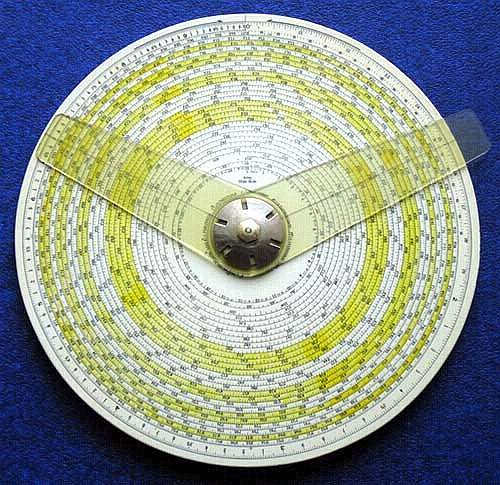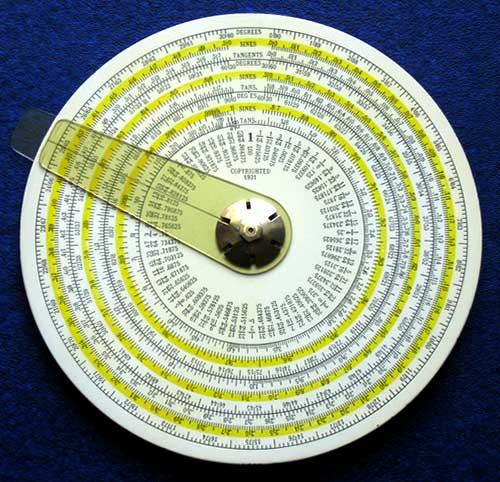previous <<==>> next

GILSON  ATLAS  CIRCULAR  SLIDE  RULE
( 8" / 21 cm Diameter )
>>>   SlideRule (*) LENGTH = 10.6 Meter = 417 Inch = 35 Feet   <<<REMARK: The ANGLE between SC- & LC-CURSOR represent the 1st FAKTOR``` S C A L E S of the CIRCULAR SLIDE RULE >> ATLAS << (1931) ******************************************************************** made by GILSON SLIDE RULE CO., Stuart, Florida / USA Front Side with 2 Cursors ( Long Cursor = LC, Short Cursor = SC ): (1) Circular X-Scale Diameter = 20 cm = 8 inch (2) 1/4 Segment Circular Radius = 97 mm = 3 7/8 inch ( labeled "sLOG" 0 .. 05 .. 10 .. 15 .. .. 95 .. 100 ) (3) Long Spiral X-Scale Length = 11 meter = 35 feet ( starts inside ... ends outside for better resolution !!!) (4) Circular lgX Scale Diameter = 68 mm = 2 3/4 inch ( labeled "cLOG" 0 .. 0.05 .. 0.1 .. 0.15 .. .. 1.0 ) (5) Coils Directory ( 1 ... 25 ) (6) List of Constants ( ... e, π, 1/π, ... ) -------------------------------------------------------------------- Back Side with a Single Cursor: (1) 3 DEGREES Circles ( 0°/90° .. 30°/60° .. 60°/30° .. 90°/0° ) (2) 3 SIN; COS Circles ( 0 ... 0.5 ... 0.866 ... 1 ) (3) 3 TAN; COT Circles ( 0 ... 0.577 ... 1.732 ... oo ) (4) Fraction-to-Decimal Conversion Table ( 1/64 ... 63/64 ) R E M A R K S : ******************************************************************** Using the ATLAS Spiral Scale with 25 turns, results may be found to 5 figures. Logarithms of numbers may be found to 5 figures too. To conserve space, instead of a parallel Spiral Log Scale with 25 turns there is only a QUARTER sLOG Scale drawn ( 1/25 ) / 4 = 0.01 to obtain the last 3 figures of the Logs ... Calculating the Length of the Spiral in the A T L A S Slide Rule ==================================================================== 25 "Rings" ; Inner Diameter = 75 mm ... 195 mm = Outer Diameter Difference = 5 mm ( Distance about 2.5 mm on each Side ) L = π * 75 + π * 80 + ... + π * 185 + π * 190 + π * 195 Is there a better way to calculate this ??? YES !!! -------------------------------------------------------------------- (As little K.F.GAUSS found out when he had to add 1 + 2 + ... + 100) L = π * ( 75 + 80 + 85 + ... + 135 + ... + 185 + 190 + 195 ) +------ sum = 270 --------+ +----------- sum = 270 --------------+ +---------------- sum = 270 --------------------+ L = π * ( 12 * 270 + 135 ) = 10602,87 WOW! = 10.6 METER How to use the A T L A S Slide Rule ==================================================================== MULTIPLICATION Example: 5 * 3 = 15 ************************************* Set the longer cursor LC on 5 and hold it. Set the shorter cursor SC on 1. Turn LC ( SC will follow, keeping the angle ) until SC is over 3. Read the result under LC. DIVISION Example: 5 / 3 = 1.666 ********************************** Set the longer cursor LC on 5 and hold it. Set the shorter cursor SC on 3. Turn LC ( SC will follow, keeping the angle ) until SC is over 1. Read the result under LC. Get a MORE PRECISE RESULT using the 11 meter ( 35 foot ) SPIRAL: ------------------------------------------------------------------- Example: 22 / 7 = 3.142857 <<== "true result" 1st Step: Make the calculation with the X-Scale on the outer ring, to get the sequence of the result-figures... = 3.14(3) < 3 digits precise > ( and under LC the expected coil number 13 ) 2nd Step: Start the calculation again, using the spiral scale to get a better result... = 3.142(8) < 4 digits precise > ( in coil 13 ) LOG Example (1): lg16 = 1.204119 <<== "true result" *********************************** "0" Step: Because the 16 has 2 digits, set = 1.____ 1st Step: Set the longer cursor LC to 16 on the circular X scale. Read the result on cLOG scale under LC: 0.20(4) = 1.20(4) < 2 digits precise > Get a MORE PRECISE RESULT using the 1/4 SEGMENT CIRCULAR sLOG: --------------------------------------------------------------- 2nd Step: Set the longer cursor LC to 16 on the SPIRAL SCALE. Read the 3rd & 4th DIGITS on sLOG under LC: = ..41(2) < 2 digits precise > Combined: = 1.2041(2) ======================= LOG Example (2): lg5 = 0.698970 <<== "true result" ********************************** "0" Step: Because the 5 has 1 digit, set = 0.____ 1st Step: Set LC to 5 on the circular X scale. Read the result on cLOG scale under LC: 0.69(8) = 0.69(8) < 2 digits precise > 2nd Step: Set LC to 5.0 on the SPIRAL SCALE. The sLOG scale is only a 1/4 circle. In this example the location of the number in the spiral is outside of the direct readability of the sLOG scale. Notice, that the COIL-DIRECTORY has STROKE (|), PERCENT (%) & PLUS (+) MARKS. These marks refer to the marks on the outer circle ( AS PARTS OF THE sLOG SCALE ) where the SC has to be PLACED. ( Observe the relative LC position in its quadrant ! ) In this example the hairline is near the (%). Set SC to mark (%) on the outer circle and turn LC ( SC will follow, keeping the angle ) until SC is over (+) on sLOG. Read the 3rd & 4th DIGITS on sLOG scale under LC: = ..89(7) < 2 digits precise > Combined: = 0.6989(7) ======================= (*) R E M A R K : Compare and A D M I R E the SIMPLICITY of the »ATLAS« CIRCULAR with the FAMOUS  » T H A C H E R « (= 30 Feet ) This GILSON Circular SlideRule was also distributed under other brand names like DIETZGEN, POST, ... Historical Remarks ... impressum: ******************************************************************** © C.HAMANN http://public.BHT-Berlin.de/hamann 06/10/08 ```